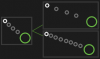# Legacy GMDraw chain on freely moving object

#### AngelofGamin

##### Member
Have you ever seen how a chain chomp works? That's basically what I'm trying to accomplish except the object can move freely around the room.

The starting point of the chain (white) should either stretch however many chain links evenly across the line between the starting chain and the object (green).

Or alternatively,

have the starting chain draw as many evenly spaced links as it needs to until it reaches the object.

Which ever one is easier to accomplish.Last edited:

#### CloseRange

##### Member
for stretching x circles:
Code:
``````var x1 = 0; // start of chain position
var y1 = 0;
var x2 = x; // end of chain position
var y2 = y;

var outR = 16; // outer radius
var inR = 8; // inner radius

var d = point_distance(x1,y1, x2, y2);
var dir = point_direction(x1, y1, x2, y2);
var dx = x1 + lengthdir_x(i*d/links, dir);
var dy = y1 + lengthdir_y(i*d/links, dir);
draw_set_color(c_white);
draw_circle(dx, dy, outR);
draw_set_color(c_black);
draw_circle(dx, dy, inR);
}``````
for making chains until they are to fit:
Code:
``````var x1 = 0; // start of chain position
var y1 = 0;
var x2 = x; // end of chain position
var y2 = y;

var padding = 4; // distance between chains
var outR = 16; // outer radius
var inR = 8; // inner radius

var d = point_distance(x1,y1, x2, y2);
var dir = point_direction(x1, y1, x2, y2);
var dx = x1 + lengthdir_x(i, dir);
var dy = y1 + lengthdir_y(i, dir);
draw_set_color(c_white);
draw_circle(dx, dy, outR, false);
draw_set_color(c_black);
draw_circle(dx, dy, inR, false);
}``````
the code is almost identical just changing how the for loop works and how distance is calculate in lengthdir_x/y

•DaMuffin and AngelofGamin

#### AngelofGamin

##### Member
for stretching x circles:
Code:
``````var x1 = 0; // start of chain position
var y1 = 0;
var x2 = x; // end of chain position
var y2 = y;

var outR = 16; // outer radius
var inR = 8; // inner radius

var d = point_distance(x1,y1, x2, y2);
var dir = point_direction(x1, y1, x2, y2);
var dx = x1 + lengthdir_x(i*d/links, dir);
var dy = y1 + lengthdir_y(i*d/links, dir);
draw_set_color(c_white);
draw_circle(dx, dy, outR);
draw_set_color(c_black);
draw_circle(dx, dy, inR);
}``````
for making chains until they are to fit:
Code:
``````var x1 = 0; // start of chain position
var y1 = 0;
var x2 = x; // end of chain position
var y2 = y;

var padding = 4; // distance between chains
var outR = 16; // outer radius
var inR = 8; // inner radius

var d = point_distance(x1,y1, x2, y2);
var dir = point_direction(x1, y1, x2, y2);
var dx = x1 + lengthdir_x(i, dir);
var dy = y1 + lengthdir_y(i, dir);
draw_set_color(c_white);
draw_circle(dx, dy, outR, false);
draw_set_color(c_black);
draw_circle(dx, dy, inR, false);
}``````
the code is almost identical just changing how the for loop works and how distance is calculate in lengthdir_x/y

All I need to do is stick this to the step event of whatever object I want a chain attached to, right?

#### CloseRange

##### Member
@AngelofGamin the draw event but yes. You can also edit it as you please.

•AngelofGamin

#### AngelofGamin

##### Member
@AngelofGamin the draw event but yes. You can also edit it as you please.
tried it and it worked pretty well, though you forgot to add the false at the end of draw circle statements on stretch

one more thing i gotta ask, say i wanted the chain to be a sprite that I personally made, how would this whole thing change?

nevermind, I just gotta use draw_sprite instead of draw_circle

Last edited:

#### CloseRange

##### Member
Yea that's all you'd also change the outR to be the size of the sprite.
And sorry I wrote the code in the forums not in game maker so not suprised I forgot that

•AngelofGamin

#### TheouAegis

##### Member
I think Chain Chomp's chains were actual objects, affected by gravity ("gravity") even. But if I ever did have the code for them, it's on my dead laptop...Basically, if i remember right, the link attached to Bow-Wow follows him if he gets too far away. Then the link next to that one follows it if it gets too far away. Then the link next to that link follows it if it gets to far away. And so forth. When Bow-Wow stops attacking, gravity kicks in and pulls Bow-Wow and the links to the ground. In other words, the links defy gravity only when Bow-Wow attacks.

•DaMuffin and AngelofGamin